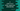# C program to print from A to Z with lower and upper case alternatively### C program to print from A to Z with lower and upper case alternatively :

In this C programming tutorial, we will learn how to print from A to Z in an alternative case for each character. For example, if the first character is A, second should be b, the third element should be C etc. That means, it will be like A b C d E f…. To solve this problem, we are going to use the below algorithm :

### The algorithm to solve it :

1. Create two variables to hold the upper and lower case A character.
2. Create one flag to detect if the lower case to print or upper case to print.
3. Run one loop to print the values each time. Print upper case once and next print lower case . Run this loop till Z is printed.
4. Inside the loop, always keep changing the value of the flag.Also, keep the character value incrementing.

### C program :

``````#include<stdio.h>
int main()
{
//1
char lowerCase = 'a';
char upperCase = 'A';
int printLowerCase = 0;

//2
while(lowerCase <= 'z' & upperCase <= 'Z'){
//3
if(printLowerCase){
printf("%c ",lowerCase);
}else{
printf("%c ",upperCase);
}
//4
printLowerCase = !printLowerCase;
lowerCase ++;
upperCase ++;
}

//5
printf("\n");
return 0;
}``````

### Explanation :

1. Create two character variables lowerCase and upperCase to store the lower and upper case value of A. Also create one integer variable printLowerCase and assign it a value 0. 0 means print the uppercase and 1 means print the lowercase letter. It will work as a flag.
2. Run one while loop. It will run till the current value is less than Z(in both lower and upper case form).
3. Inside the loop, check the value of the flag.If it is 1, print the current uppercase character, else print the current lower case character.
4. Change the value of the flag. If it is 1, make it 0. If it is 0, make it 1. Also, increment the current character. (both lower and upper case character) by one. Means, lowerCase will hold the next lowercase character and upperCase will hold the next uppercase character.
5. After the loop is completed, print one new line.

### Output :

``A b C d E f G h I j K l M n O p Q r S t U v W x Y z``